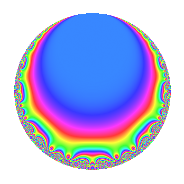# Properties

 Label 28.3.hLevel 28 Weight 3 Character orbit h Rep. character $$\chi_{28}(5,\cdot)$$ Character field $$\Q(\zeta_{6})$$ Dimension 2 Newforms 1 Sturm bound 12 Trace bound 0

# Related objects

## Defining parameters

 Level: $$N$$ = $$28 = 2^{2} \cdot 7$$ Weight: $$k$$ = $$3$$ Character orbit: $$[\chi]$$ = 28.h (of order $$6$$ and degree $$2$$) Character conductor: $$\operatorname{cond}(\chi)$$ = $$7$$ Character field: $$\Q(\zeta_{6})$$ Newforms: $$1$$ Sturm bound: $$12$$ Trace bound: $$0$$

## Dimensions

The following table gives the dimensions of various subspaces of $$M_{3}(28, [\chi])$$.

Total New Old
Modular forms 22 2 20
Cusp forms 10 2 8
Eisenstein series 12 0 12

## Trace form

 $$2q$$ $$\mathstrut +\mathstrut 3q^{3}$$ $$\mathstrut +\mathstrut 3q^{5}$$ $$\mathstrut -\mathstrut 14q^{7}$$ $$\mathstrut -\mathstrut 6q^{9}$$ $$\mathstrut +\mathstrut O(q^{10})$$ $$2q$$ $$\mathstrut +\mathstrut 3q^{3}$$ $$\mathstrut +\mathstrut 3q^{5}$$ $$\mathstrut -\mathstrut 14q^{7}$$ $$\mathstrut -\mathstrut 6q^{9}$$ $$\mathstrut -\mathstrut 15q^{11}$$ $$\mathstrut +\mathstrut 6q^{15}$$ $$\mathstrut +\mathstrut 51q^{17}$$ $$\mathstrut +\mathstrut 27q^{19}$$ $$\mathstrut -\mathstrut 21q^{21}$$ $$\mathstrut +\mathstrut 9q^{23}$$ $$\mathstrut -\mathstrut 22q^{25}$$ $$\mathstrut -\mathstrut 12q^{29}$$ $$\mathstrut -\mathstrut 21q^{31}$$ $$\mathstrut -\mathstrut 45q^{33}$$ $$\mathstrut -\mathstrut 21q^{35}$$ $$\mathstrut -\mathstrut 31q^{37}$$ $$\mathstrut +\mathstrut 24q^{39}$$ $$\mathstrut +\mathstrut 20q^{43}$$ $$\mathstrut -\mathstrut 18q^{45}$$ $$\mathstrut +\mathstrut 75q^{47}$$ $$\mathstrut +\mathstrut 98q^{49}$$ $$\mathstrut +\mathstrut 51q^{51}$$ $$\mathstrut +\mathstrut 57q^{53}$$ $$\mathstrut +\mathstrut 54q^{57}$$ $$\mathstrut -\mathstrut 141q^{59}$$ $$\mathstrut -\mathstrut 141q^{61}$$ $$\mathstrut +\mathstrut 42q^{63}$$ $$\mathstrut -\mathstrut 24q^{65}$$ $$\mathstrut +\mathstrut 49q^{67}$$ $$\mathstrut -\mathstrut 252q^{71}$$ $$\mathstrut -\mathstrut 45q^{73}$$ $$\mathstrut -\mathstrut 66q^{75}$$ $$\mathstrut +\mathstrut 105q^{77}$$ $$\mathstrut +\mathstrut 73q^{79}$$ $$\mathstrut -\mathstrut 9q^{81}$$ $$\mathstrut +\mathstrut 102q^{85}$$ $$\mathstrut -\mathstrut 18q^{87}$$ $$\mathstrut +\mathstrut 99q^{89}$$ $$\mathstrut -\mathstrut 21q^{93}$$ $$\mathstrut +\mathstrut 27q^{95}$$ $$\mathstrut +\mathstrut 180q^{99}$$ $$\mathstrut +\mathstrut O(q^{100})$$

## Decomposition of $$S_{3}^{\mathrm{new}}(28, [\chi])$$ into irreducible Hecke orbits

Label Dim. $$A$$ Field CM Traces $q$-expansion
$$a_2$$ $$a_3$$ $$a_5$$ $$a_7$$
28.3.h.a $$2$$ $$0.763$$ $$\Q(\sqrt{-3})$$ None $$0$$ $$3$$ $$3$$ $$-14$$ $$q+(1+\zeta_{6})q^{3}+(2-\zeta_{6})q^{5}-7q^{7}-6\zeta_{6}q^{9}+\cdots$$

## Decomposition of $$S_{3}^{\mathrm{old}}(28, [\chi])$$ into lower level spaces

$$S_{3}^{\mathrm{old}}(28, [\chi]) \cong$$ $$S_{3}^{\mathrm{new}}(14, [\chi])$$$$^{\oplus 2}$$